# Stochastic processes, prediction of

(diff) ← Older revision | Latest revision (diff) | Newer revision → (diff)

extrapolation of stochastic processes

The problem of estimating the values of a stochastic process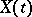in the future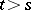from its observed values up to the current moment of time. Usually one has in mind the extrapolation estimator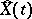,, for which the mean-square erroris minimal over all estimators based on the past values of the process up to the moment(the prediction is called linear if one restricts consideration to linear estimators).

One of the problems posed and solved was that of linear prediction of a stationary sequence. This problem is analogous to the following: In the space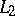of square-integrable functions on the interval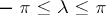one has to find the projection of the function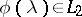onto the subspace generated by the functions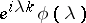,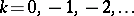. This problem has been greatly generalized in the theory of stationary stochastic processes (cf. Stationary stochastic process). One application is the problem of predicting stochastic processes arising from the systemwhereis a linear differential operator of orderand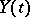,, is a white noise process. The optimal prediction,, given the values ofat the times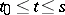and the initial values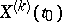,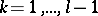, independent of white noise, is obtained by solving the corresponding equationwith initial conditions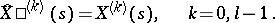For systems of stochastic differential equations, the problem of predicting some components given the values of other observed components reduces to the extrapolation of the corresponding stochastic equations.

For references see Stochastic processes, interpolation of.